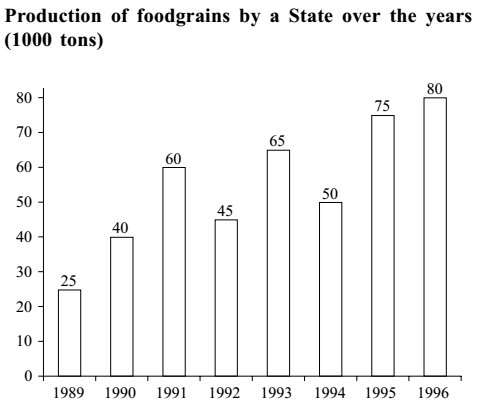#### Data Interpretation

Direction: Study the following graph carefully and answer the questions given below it.1. The average production of 1990 and 1991 was exactly equal to the average production of which of the following pairs of years?
1. 1991 and 1992
2. 1992 and 1994
3. 1993 and 1994
4. 1994 and 1995
5. None of these
##### Correct Option: E

As per given graph in question,
The average production of 1990 and 1991 = (40 + 60)/2 = 100/2 = 50
The average production of 1991 = 60
Solve one by one option.
(A) The average production of 1991 and 1992 = (60 + 45)/2 = 105/2 = 52.5
(B) The average production of 1992 and 1994 = (45 + 50)/2 = 95/2 = 47.5
(C) The average production of 1993 and 1994 = (65 + 50)/2 = 115/2 = 57.5
(D) The average production of 1994 and 1995 = (50 + 75)/2 = 125/2 = 62.5

All the given option doesn't have average production of 50. So answer will be none of these.